Thank you for visiting nature.com. You are using a browser version with limited support for CSS. To obtain the best experience, we recommend you use a more up to date browser (or turn off compatibility mode in Internet Explorer). In the meantime, to ensure continued support, we are displaying the site without styles and JavaScript.

# Rashba Spin-Orbit Anisotropy and the Electric Field Control of Magnetism

## Abstract

The control of the magnetism of ultra-thin ferromagnetic layers using an electric field, rather than a current, has many potential technologically important applications. It is usually insisted that such control occurs via an electric field induced surface charge doping that modifies the magnetic anisotropy. However, it remains the case that a number of key experiments cannot be understood within such a scenario. Much studied is the spin-splitting of the conduction electrons of non-magnetic metals or semi-conductors due to the Rashba spin-orbit coupling. This reflects a large surface electric field. For a magnet, this same splitting is modified by the exchange field resulting in a large magnetic anisotropy energy via the Dzyaloshinskii-Moriya mechanism. This different, yet traditional, path to an electrically induced anisotropy energy can explain the electric field, thickness and material dependence reported in many experiments.

## Introduction

The possibility of controlling the magnetic anisotropy of thin ferromagnetic films using a static electric field E is of great interest since it can potentially lead to magnetic random access memory (MRAM) devices which require less energy than spin-torque-transfer random access memory STT-MRAM1,2,3,4,5,6,7. Thin magnetic films with a perpendicular magnetic anisotropy (PMA) are important for applications8,9. That an interfacial internal electric field might be used to engineer such a PMA is also of great interest. Experiment10,11 has indeed shown that such a PMA might, in turn, be modified by an externally applied electric field, however the data is usually interpreted in terms of changes to the electronic contribution to magnetic anisotropy due to the surface doping induced by the applied electric field3,11,12.

The theory of the field-induced changes of the magnetic anisotropy reflecting surface doping is invariably developed in terms of band theory13,14,15,16,17. The results for both the bulk and thin films can be adequately understood in terms of second order perturbation theory18 in which the relevant matrix elements of the spin-orbit interaction are between full and empty states. Large contributions come from regions where different d-bands (almost) cross. That such crossings should be close to the Fermi surface leads to a strong doping dependence in such theories. Nakamura et al.19 pointed out that the strong negative applied field dependence of the PMA for an isolated mono-layer (ML) of Fe(001) arises directly from band splitting rather than from doping. In this case, an E perpendicular to the film breaks reflection symmetry causing a large spin-orbit splitting of d-levels near the Fermi surface. As will be explain below, despite these important theoretical developments, a clear explanation of a number of key experiments is still lacking.

Here we develop a simple analytic theory for the existence and electrical control of the PMA based upon the Rashba spin-orbit interaction20,21,22 and the single band Stoner model of magnetism. We exhibit the somewhat delicate, but very interesting, competition between the Rashba spin-orbit fields and the exchange interaction, reflecting electron correlations. This theory can potentially lead to a very large magnetic anisotropy arising from the internal electric fields Eint which exist at, e.g., ferromagnetic/metal and ferromagnetic/oxide insulator interfaces but modified by the addition of an applied electric field Eext. There is a Rashba splitting of the band structure leading to a quadratic, (Eint + Eext)2, contribution to the magnetic anisotropy, contrasting with a linear in Eext doping effect.

## Results

### Model

This comprises a band Stoner model with the Rashba interaction added23: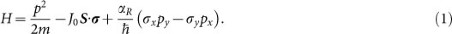where p is the electron momentum operator, S the order parameter, σ the Pauli matrices and αR = soE the Rashba parameter proportional to ηso, which characterises the spin-orbit coupling. The electric field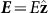is taken to be perpendicular to the plane of the system andis perpendicular toand makes an angle θ to the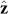-direction, as in Fig. 1(a).

### Illustrative non-magnetic example

Consider the Rashba effect in non-magnetic two dimensional electron gases or surface states on noble metals, e.g., a surface state of Au. As shown in the methods, the single particle energy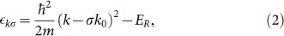as the spin quantum number σ = ±1. The momentum shift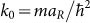and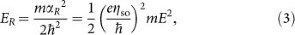identified in the inset of Fig. 1(b), reflects the single particle energy gain relative to zero electric field, i.e., E = 0 and hence αR = 0. For the three dimensional problem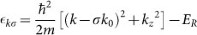and there is no equivalent momentum shift in kz. For the surface state of Au, ER ≈ 3.5 meV24,25 exemplifying the energy scale.

The study of such surface states and differences in chemical potentials, also helps set the scale for E. Between a metal and the vacuum, or dielectric, the E ~ 10 V/nm near the Cu (100) surface26, reflects the electron image potential. For the Ag/Cu(111) system27 an E of similar magnitude occurs at the metal interface. The difference in the (111) chemical potentials28 of Cu(4.96 eV) and Ag(4.74 eV) is reflected by a potential increase from Cu to Ag. The chemical potential of Au(5.31 eV) implies a similar potential change and E between Cu and Au, but of the opposite sign. It is the s-electrons which penetrate the core and determine the spin-orbit parameter ηso. The example Cu/Ag/Au therefore illustrates the higher/lower potential of these s-electrons in the second/third transition series relative to the similar electrons in the 3d elements.

There are many experiments24,25,29,30,31 which put in evidence the Rashba splitting in two dimensional electron gases, surface states of noble metals, bulk layered systems and e.g., of a surface state of ferromagnetic Yb.

### Magnetic case - origin of the magnetic anisotropy energy

In the methods it is shown the θ dependent single particle energy, i.e., the equivalent of Eq. (2) for the magnetic case is: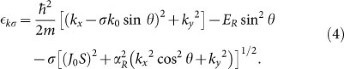The direction of the now θ dependent momentum shift changes sign as the spin index σ = ±1. These shifts also change sign with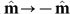for a given σ. This “magnetic Rashba splitting” with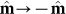is observed for the surface state of Yb31.

Also in the methods the Dzyaloshinskii-Moriya (DM) and pseudo-dipolar (PD) contributions to the magnetic anisotropy are highlighted by contrasting the perpendicular and parallel orientations of order parameter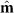to the plane.

Assuming (J0S)2 > (αRkx)2 and retaining the θ-dependent terms up to the order of E2 in (4), we obtained our principal result: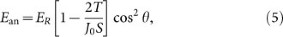for the magnetic anisotropy energy, with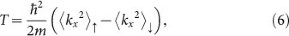where 〈 〉 denotes an average over the Fermi sea (see methods). The Rashba spin-orbit interaction produces a uni-axial anisotropy energy which, as in the Dzyaloshinskii-Moriya theory32,33,34,35, comprises a direct second order in E easy plane pseudo-dipolar interaction and an indirect contribution proportional to E2/J0S reflecting the competition between the first order in E, Rashba-Dzyaloshinskii-Moriya and exchange fields.

### Competition between the Dzyaloshinskii-Moriya and pseudo-dipolar contributions

Clearly Eq. (5) implies an E2 dependent PMA results if T > J0S/2, i.e., when the DM is larger than the PD term. Taken literally, the Stoner model Eq. (1), with its quadratic dispersion, predicts the ratio of the DM and PD contributions to the PMA. The result, (see methods), depends upon the spatial dimension. In two dimensions the PD and DM terms cancel although higher order terms (OR4)) lead to a PMA while in three dimensions the DM term is −(4/5) ER cos2 θ and an in-plane magnetisation is favoured. Lastly, a two dimensional system with a highly anisotropic conductivity might be modelled as a series of parallel one dimensional chains. For chains the DM contribution −(4/3) ER cos2 θ which dominates the PD energy ER cos2 θ, appropriate whenis in-plane and perpendicular to the chains. Corresponding to the hardest axis, whenin-plane but rather parallel to the chains, there is neither a DM or PD contribution to the magnetic anisotropy energy. There is thereby a predicted electric field dependence of the in-plane anisotropy as seen in early experiments3, given the large compressive strain that arises in these experiments.

However, for the real problem of 3d magnets, a quadratic dispersion is not at all realistic and the crystal potential V(r) must be accounted for, see methods. For 3d elements the wavefunction ψ is well localised within the atomic sphere and the averages, e.g.,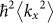and hence T, are very much increased as compared to the above naïve estimates. In reality, the DM contribution will invariably lead to a PMA.

## Discussion

The resulting anisotropy energy can be very large. The work already cited24,25,26,27 on conducting but non-magnetic materials helps set the scales. The value of the scaling prefactor ER in Eq. (5) for the surface state of Au is ~ 3.5 meV or about 35 T in magnetic field units and very much larger than the typical ~ 1 T demagnetising field. If a Au film is polarised by contact with an ultra-thin ferromagnet, the second factor, 2T/J0S, in Eq. (5) for the field inside a Au surface layer can be quite large ~ 5 leading to a PMA and indeed ultra-thin Fe on Au does have such a PMA36,37. Ultra-thin ferromagnetic films in contact with, e.g., Ru, Pd, Pt and Ta, etc., also are found to have a PMA6,12,38,39.

Schematically shown in Fig. 2(a) is the potential seen by electrons in a free standing ultrathin ferromagnetic film. At the surface, the potential reflects an electron's image charge but reaches the vacuum level within a few atomic spacing. As already discussed, this results in a finite large electric field E ~ 10 V/nm at each surface but in opposite senses. Assuming an appreciable spin-orbit coupling in the interface region, this results, in turn, in a Rashba field BR which also changes sign between the two surfaces for a given momentum. Thus, for a perfectly symmetric film, the ferromagnetically polarised electrons see no average field BR. This symmetry can be broken by the application of an external electric field as shown in Fig. 2(b). The electric field is increased at one surface and decreased at the other doubling the net effect. In contrast, for this same symmetric situation, the surface charges are opposite and doping effects must cancel. Experimentally applied fields of 1 V/nm are relatively easy to achieve implying a ~ 10% change in the surface anisotropy. Experiments40 with a 1.5 nm Fe80B20 sandwiched between two MgO layers are perhaps closest to this situation although the thickness 1.5 nm and 2.5 nm of these layers are not equal. Roughly consistent with our estimate [see Fig. 2(c), case(i)], there is40 an ~ 15% symmetric contribution to the magnetic anisotropy for an applied voltage of 2 V.

Clearly the intrinsic Rashba field BR is modified when the materials adjacent to a 3d ferromagnet (F) are different. In a number of experiments an insulator I, often MgO, lies to one side and a normal metal (N), e.g., Au, Pt, Pd, Ta, or Ru, “cap” completes a tri-layer system. The potential, Figs. 2(d) and (e), will increase in passing from Fe to MgO but in passing from the F to N-layer the potential will either increase, Fig. 2(d), or decrease, Fig. 2(e). As discussed above, the relevant potential would be expected to increase, Fig. 2(d), in passing from a 3d element, e.g., Co, Fe, Ni, or Cu, to a 4d transition metal such as Ru, Pd, Ag but decrease, Fig. 2(e), for the 5d elements, e.g., Au, Pt, Ta. The latter case is particularly favourable since the intrinsic Rashba fields have the same sense and add. In addition, the 5d elements have a larger spin-orbit coupling, resulting in a larger αR and hence are more likely to produce a sizeable PMA. If the electric field decreases at the FI interface, the average Rashba field increases in the first case [Fig. 2(f)] when the effects of the surfaces tend to cancel and, as illustrated in Fig. 2(g), decreases in the second case when the inverse is true [see Fig. 2c, case (ii) and (iii)]. Experiment12 indeed shows an opposite field dependence for such systems with Pd(4d) and Pt(5d) N-layers. That the sign of the electric field contribution to the PMA reflects the N-layer whereas the field is applied to the opposite surface between F and I supports the current Rashba model. This is in stark contrast with the popular surface doping model3,12, for which the effects of surface doping are limited by the (possibly magnetically modified) Fermi-Thomas screening length. In reality12, the screening length is estimated to be much less than 1 nm and much too short for there to be an appreciable doping effect of the Pt or Pd layers that are typically distant by a few nanometers.

Simulating a large applied electric field E, the required asymmetry might be controlled in NFN tri-layers by varying in a systematic manner, at the mono-layer level, the thickness of one of the normal metal layers and by using metals with different spin-orbit couplings. In reality the effect of the substrate transmitted to and through, the bottom normal metal will imply an asymmetry even for largish N-layer thickness. Indeed the PMA surface term for Au/Fe(110)/Au(111) structures does show an non-monotonic dependence on the top Au layer thickness36. Experiments37 for Fe layers on vicinal Ag(001) and Au(001) surfaces and which undergo a symmetry breaking (5 × 20) surface reconstruction manifest an in-plane surface term reflecting this broken symmetry and which is larger for Au, with its stronger spin-orbit coupling, than for Ag.

It is predicted that the surface coercivity field Hc is proportional to (Eint + Eext)2 where Eint is the internal electric field corresponding to the zero-bias Rashba contribution to the anisotropy. Such a non-linear field dependence is observed, e.g., for the in-plane contribution for a (Ge,Mn)As/ZrO2 surface3. In other experiments10 with CoFeB/MgO/CoFeB structures there is qualitative difference between the E dependence of the anisotropy field Hc of the, “top” and “bottom”, CoFeB layers of this three layer structure, even when they have similar thicknesses. The bottom layer has a larger Hc and is roughly linear while Hc becomes highly non-linear as Hc → 0 as would be expected as Eext → −Eint.

The most direct experimental test of the model is the observation of the band splittings for a model Rashba system with a variable contact with an itinerant ferromagnet. This can result in giant magnetic anisotropy (GMA) energies. For example an ER ~ 100 meV (or ~ 1000 T) is reported in angle-resolved photoemission spectroscopy (ARPES) measurements41 on bulk BiTeI. For a thin film of this, or similar material, in contact with an itinerant ferromagnet such as Fe, a suitable exchange splitting J0S, tuned to the order of ER, might be induced and a GMA will result. ARPES performed as a function of the direction of the magnetisation m might determine both ER and the momentum dependence of the exchange splitting leading to estimates of both the PM and DM contributions and which might be directly compared with magnetisation and magnetic resonance measurements. The electrical control of such a GMA has evident important application for non-volatile memory applications. There are clearly many more complicated embodiments of such a device.

In conclusion, it is suggested that the Rashba magnetic field due to the internal electric field in the surface region of an ultra-thin ferromagnet can make an important contribution to the perpendicular magnetic anisotropy. Such surface fields might be modified by application of an applied electric field. Since the internal fields at two surfaces tend to cancel, an asymmetry between the surfaces is important. Such an asymmetry is caused by different metal and insulator caping layers. These ideas are consistent with a large number of experiments.

## Methods

### The non-magnetic case

This corresponds to Eq. (1) with J0 = 0. It is solved by taking the axis of quantisation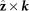to be perpendicular to the in-plane k as in Fig. 1(b). The eigenstates are eik·r|s〉 and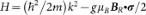, where the Rashba magnetic field in energy units is defined as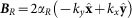, with μB the Bohr magneton and g the g-factor, leaving the spin state |s〉 to be determined. There are two concentric Fermi surfaces. The energy splitting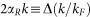, where Δ is the value for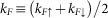, with kF↑,↓ the Fermi wave number for the spin up/down (σ = ±1) band. For the surface state of Au, Δ ≈ 110 meV while EF ≈ 420 meV giving the ER ≈ 3.5 meV cited in the text. The magnetic case. The full Eq. (1) is solved by defining axes such that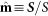lies in the yz-plane and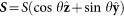. The total field, which defines the axis of quantisation,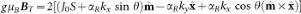. It is assumed that, for a 3d ferromagnet J0S ~ 0.5–1.0 eV and BBR < J0S, i.e., the Rashba is smaller than the exchange splitting. To second order in BBR,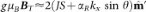where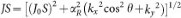and wherediffers in direction fromby a small angle δ where tan δ ≈ αR(kx2 cos2θ + ky2)1/2/J0S. The linear in kx term, αRkx sin θ, causes a shift in Fermi sea to give the the single particle energy Eq. (4).

With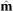perpendicular to the plane, i.e.,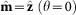, the exchange and Rashba fields are orthogonal and hence the net energy for a single electron Eq. (4) is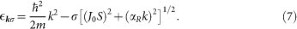The axis of quantisation is tilted by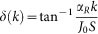away from the z-axis as shown in Fig. 1(c). The σ = ±1 electrons gain/lose an energy that is even in E. This arises from the competition of the Rashba field, perpendicular to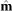, with the exchange field. Such a competition generates a second order in E contribution to the magnetic anisotropy and is identified with the Dzyaloshinskii-Moriya (DM) mechanism32,33,34,35.

Now takeparallel to the y-axis, i.e.,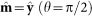. The y-component of BR is parallel to the exchange field and is combined with the kinetic energy. The Fermi sea is shifted along the x-axis and lowered by ER as shown in Fig. 1(d). This energy gain corresponds to a pseudo-dipolar (PD) contribution to anisotropy energy35 which favours an in-plane magnetisation. On the other hand, the x-component of BR, which is perpendicular to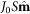, gives rise to a correction to the effective exchange field. The direction of the moment tilts away from the y-axis in the direction perpendicular to the wave vector by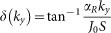as shown in Fig. 1(d). The single particle energy, Eq. (4), is now,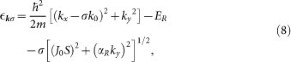where the shift k0 is the same as in Eq. (2) but only along the x-axis.

The effective exchange field in Eq. (8) is smaller than that in Eq. (7) due to the absence of a kx2 term. This indicates that the overall DM contribution favours a perpendicularwhile the PD term favours an in-plane. This exchange field changes sign with σ = ±1.

### Evaluation of the Dzyaloshinskii-Moriya and pseudo-dipolar contributions

Needed for T in Eq. (5) are the Fermi sea averages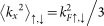and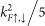, determined analytically, for quadratic dispersion, in one, two and three dimensions respectively. For an isotropic system these averages are related to J0S via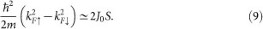which determines the ratio 2T/J0S in the principal result, Eq. (5), given in the text.

### Role of the crystal potential

The effects of the crystal potential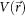are exhibited by considering a wave function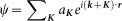which is a linear combination of plane waves, where K are the reciprocal lattice vectors and the aK are determined by V (r). While not convenient for 3d electrons, at least in principle, such an expansion in the true, rather than crystal, momentum states is always possible. The PD contribution, ER cos2 θ is independent of the momentum k + K. However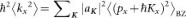, where 〈 〉BZ is the average over the first Brillouin zone. For 3d electrons, the average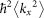and hence T, are dominated by the aK for largish K. It follows T is significantly increased with the consequences discussed in the text.

## References

• Eerenstein, W., Mathur, N. D. & Scott, J. F. Multiferroic and magnetoelectric materials. Nature 442, 759–765 (2006).

• Chiba, D., Yamanouchi, M., Matsukura, F. & Ohno, H. Electrical manipulation of magnetization reversal in a ferromagnetic semiconductor. Science 301, 943–945 (2003).

• Chiba, D. et al. Magnetization vector manipulation by electric fileds. Nature 455, 515–518 (2008).

• Chu, Y.-H. et al. Electric-field control of local ferromagnetism using a magnetoelectric multiferroic. Nat. Mater. 7, 478–482 (2008).

• Stolichnov, I. et al. Non-volatile ferroelectric control of ferromagnetism in (Ga,Mn)As. Nat. Mater. 7, 464–467 (2008).

• Weisheit, M. et al. Ferromagnets Electric Field-Induced Modification of Magnetism in Thin-Film Ferromagnets. Science 315, 349–351 (2007).

• Tsymbal, E. Y. Electric toggling of magnets. Nat. Mater. 11, 12–13 (2012).

• Emori, S. et al. Current-driven dynamics of chiral ferromagnetic domain walls. Nat. Mater. 12, 611 (2013).

• Ryu, K.-S., Thomas, L., Yang, S.-H. & Parkin, S. Chiral spin torque at magnetic domain walls. Nature Nanotech. 8, 527–533 (2013).

• Wang, W-G., Li, M., Hageman, S. & Chien, C. L. Electric-field-assisted switching in magnetic tunnel junctions. Nat. Mater. 11, 64–68 (2012).

• Maruyama, T. et al. Large voltage-induced magnetic anisotropy change in a few atomic layers of iron. Nature Nanotech. 4, 158–161 (2009).

• Fowley, C. et al. Electric field induced changes in the coercivity of a thin-film ferromagnet. J. Phys. D: Appl. Phys. 44, 305001 (2011).

• Kyuno, K., Ha, J. G., Yamamoto, R. & Asano, S. First-principle calculation of the magnetic anisotropy energies of Ag/Fe(001) and Au/Fe(001) multilayers. J. Phys. Soc. Jpn. 65, 1334–1339 (1996).

• Duan, C.-G., Jaswal, S. S. & Tsymbal, E. Y. Predicted magnetoelectric effect in Fe/BaTiO3 multilayers: ferroelectric control of magnetism. Phys. Rev. Lett. 97, 047201 (2006).

• Niranjan, M. K., Duan, C. G., Jaswal, S. S. & Tsymbal, E. Y. Electric field effect on magnetization at the Fe/MgO(001) interface. Appl. Phys. Lett. 96, 222504 (2010).

• Nakamura, K. et al. Role of an interfacial FeO layer in the electric-field-driven switching of magnetocrystalline anisotropy at the Fe/MgO interface. Phys. Rev. B 81, 220409(R) (2010).

• Tsujikawa, M. et al. A comparative ab initio study on electric-field dependence of magnetic anisotropy in MgO/Fe/Pt and MgO/Fe/Au films. J. Appl. Phys. 109, 07C107 (2011).

• Wang, D. S., Wu, R. & Freeman, A. J. First-principles theory of surface magnetocrystalline anisotropy and the diatomicpair model. Phys. Rev. B 47, 14932–14947 (1993).

• Nakamura, K. et al. Giant Modification of the Magnetocrystalline Anisotropy in Transition-Metal Monolayers by an External Electric Field. Phys. Rev. Lett. 102, 187201 (2009).

• Rashba, E. I. Properties of semiconductors with an extremum loop. 1. Cyclotron and combinational resonance in a magnetic field perpendicular to the plane of the loop. Sov. Phys. Solid State 2, 1109–1122 (1960).

• Casella, R. C. Toroidal energy surfaces in crystals with wurtzite symmetry. Phys. Rev. Lett. 5, 371–373 (1960).

• Bychkov, Y. A. & Rashba, E. I. Properties of a 2D electron gas with lifted spectral degeneracy. JETP Lett. 39, 78–81 (1984).

• Manchon, A. & Zhang, S. Theory of spin torque due to spin-orbit coupling. Phys. Rev. B 79, 094422 (2009).

• LaShell, S., McDougall, B. A. & Jensen, E. Spin splitting of Au(111) surface stateband observed with angle resolved photoelectron spectroscopy. Phys. Rev. Lett. 77, 3419–3422 (1996).

• Hoesch, M. et al. Spin structure of the Shockley surface state on Au(111). Phys. Rev. B 69, 241401 (2004).

• Höfer, U. et al. Time-Resolved Coherent Photoelectron Spectroscopy of Quantized Electronic States on Metal Surfaces. Science 277, 1480–1482 (1997).

• Bejan, D. Calculated surface, image and quantum well states in Ag/Cu(111) system. Physica B: Condens. Matter Phys. 424, 32–38 (2013).

• Michaelson, H. B. The work function of the elements and its periodicity. J. Appl. Phys. 48, 4729–4733 (1977).

• Ast, C. R. et al. Giant spin splitting through surface alloying. Phys. Rev. Lett. 98, 186807 (2007).

• Gierz, I. et al. Silicon surface with giant spin splitting. Phys. Rev. Lett. 103, 046803 (2009).

• Krupin, O. et al. Rashba effect at the surfaces of rare-earth metals and their monoxides. New J. Physics 11, 013035 (2009).

• Dzyaloshinskii, I. E. A thermodynamic theory of weak ferromagnetism of antiferromagnetics. J. Chem. Solids 4, 241–255 (1958).

• Moriya, T. Anisotropic Superexchange Interaction and Weak Ferromagnetism. Phys. Rev. 120, 91–98 (1960).

• Moriya, T. New Mechanism of Anisotropic Superexchange Interaction. Phys. Rev. Lett. 4, 228–230 (1960).

• Maekawa, S. et al. Physics of Transition Metal Oxides. (144 [1–36]), (Springer Series in Solid-State Sciences, 2004).

• Chagpert, C. et al. Magnetic anisotropy and interlayer exchange coupling in Fe(110)/Au(111) ultrathin films. J. Magn. Magn. Mater. 148, 165–166 (1995).

• Lee, T. et al. In-plane magnetic anisotropies in Fe films on vicinal Ag(001) and Au(001) surfaces. J. Appl Phys. 85, 4964–4966 (1999).

• Shiota, Y. Opposite signs of voltage-induced perpendicular magnetic anisotropychange in CoFeBjMgO junctions with different underlayers. Appl. Phys. Lett. 103, 082410 (2013).

• Kanai, S. et al. Electric field-induced magnetization reversal in a perpendicular-anisotropy CoFeB-MgO magnetic tunnel junction. Appl. Phys. Lett. 101, 122403 (2012).

• Nozaki, T. et al. Voltage-Induced Magnetic Anisotropy Changes in an Ultrathin FeB Layer Sandwiched between Two MgO Layers. Appl. Phys. Express 6, 073005 (2013).

• Ishizaka, K. et al. Giant Rashba-type spin splitting in bulk BiTeI. Nat. Mater. 10, 521–526 (2011).

## Acknowledgements

This work is partly supported by KAKENHI (No. 24740247) from MEXT, Japan.

## Author information

Authors

### Contributions

S.E.B. identified the problem. S.E.B., J.I. and S.M. performed the analytical calculations, analysed the data and wrote the manuscript. S.E.B. and J.I. devised and prepared the figures. All authors reviewed the manuscript.

## Ethics declarations

### Competing interests

The authors declare no competing financial interests.

## Rights and permissions

Reprints and Permissions

Barnes, S., Ieda, J. & Maekawa, S. Rashba Spin-Orbit Anisotropy and the Electric Field Control of Magnetism. Sci Rep 4, 4105 (2014). https://doi.org/10.1038/srep04105

• Accepted:

• Published:

• DOI: https://doi.org/10.1038/srep04105

• ### Route towards efficient magnetization reversal driven by voltage control of magnetic anisotropy

• Roxana-Alina One
• Hélène Béa
• Coriolan Tiusan

Scientific Reports (2021)

• ### Superconductivity assisted change of the perpendicular magnetic anisotropy in V/MgO/Fe junctions

• César González-Ruano
• Diego Caso

Scientific Reports (2021)

• ### Dzyaloshinskii–Moriya interaction in noncentrosymmetric superlattices

• Woo Seung Ham
• Teruo Ono

npj Computational Materials (2021)

• ### Electric-field-driven octahedral rotation in perovskite

• Wonshik Kyung
• Choong H. Kim
• Changyoung Kim

npj Quantum Materials (2021)

• ### Spintronic devices: a promising alternative to CMOS devices

• Prashanth Barla
• Vinod Kumar Joshi
• Somashekara Bhat

Journal of Computational Electronics (2021)